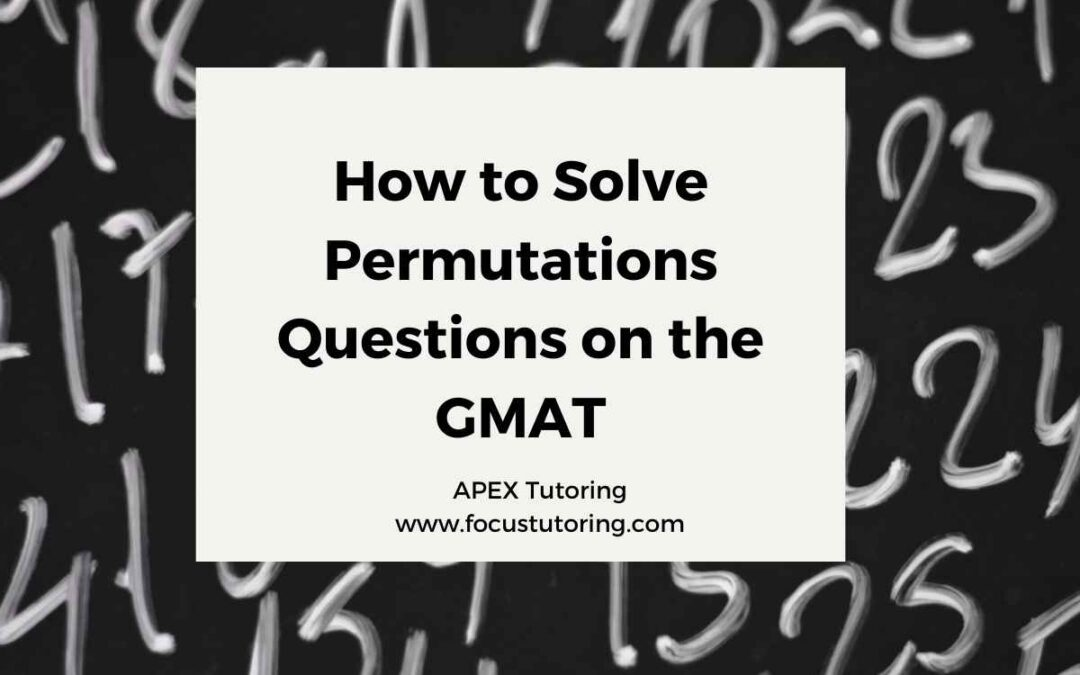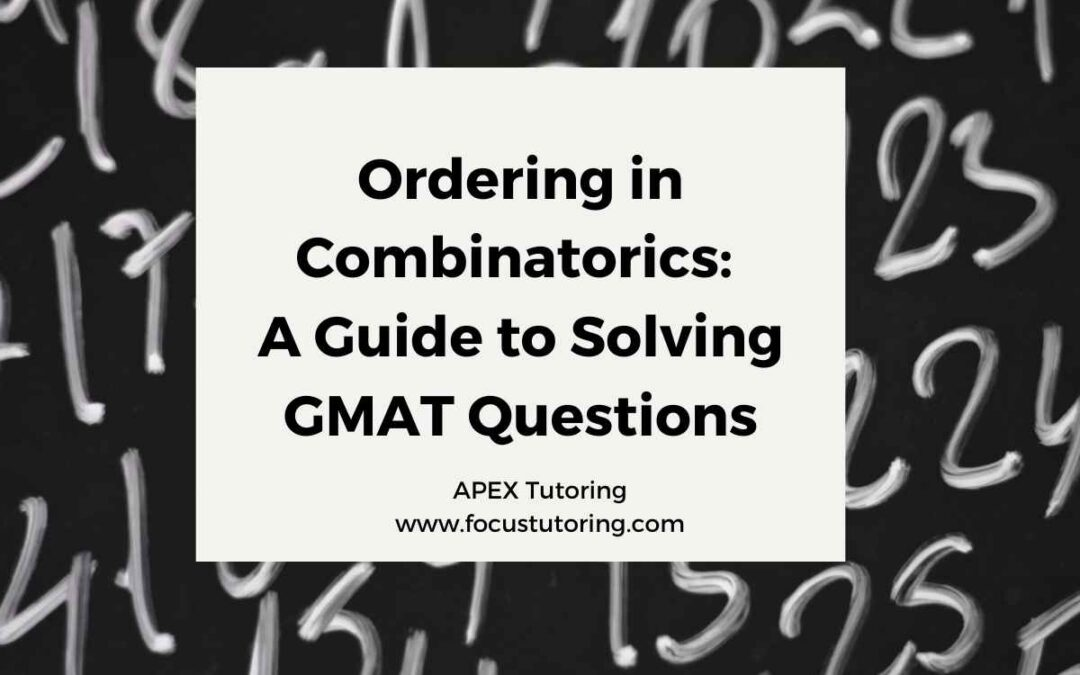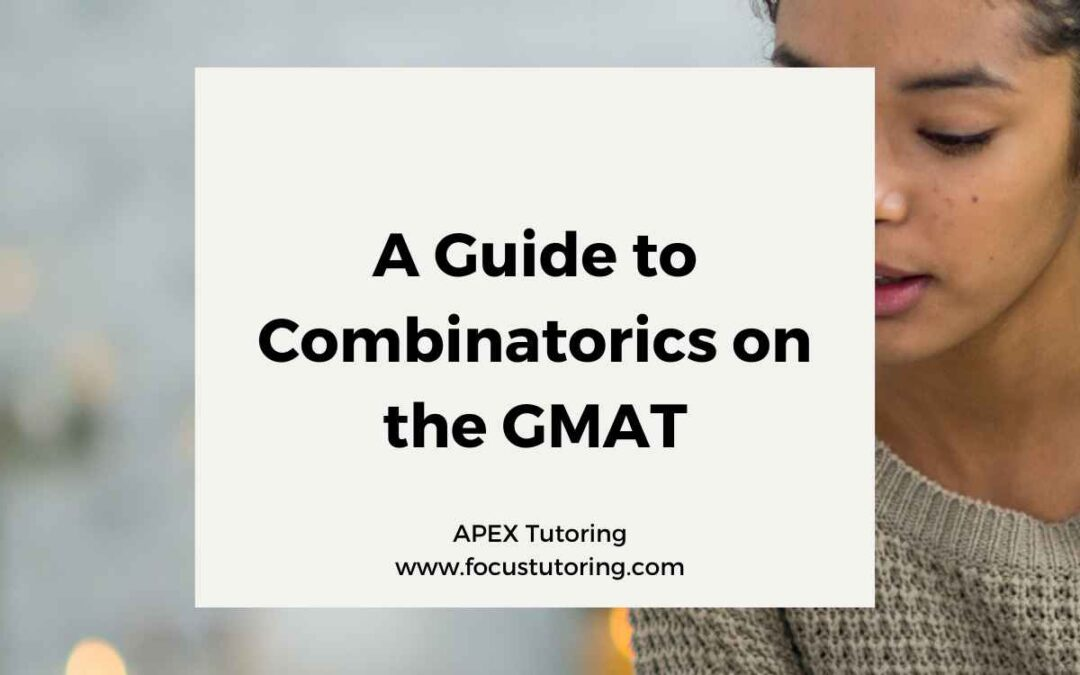## How to Solve Permutations Questions on the GMAT

Permutations are a type of combinatorics task that is often tested on the GMAT. In a permutations question, you are asked to find the number of ways to order a group of elements. The order of the elements matters in permutations questions, so the number of possible...## Ordering in Combinatorics: A Guide to Solving GMAT Questions

What is an Ordering Task? Ordering tasks are a common type of question on the GMAT. In an ordering task, you are asked to determine the number of ways to order a set of objects. The number of ways to order n different objects is n!, or n factorial. The best way to...## A Guide to Combinatorics on the GMAT

Every combinatorics question on the GMAT involves one or more of three fundamental combinatorics tasks: ordering, permutations, and combinations. This article will introduce the three tasks, and each of the three following articles will explain a task intuitively to...## GMAT Quant Syllabus 2022-2023

We know what you’re thinking: math is a scary subject and not everyone can excel at it. And now with the GMAT the stakes are much higher, especially because there is a whole section dedicated to math that you need to prepare for in order to guarantee a good score....## GMAT Rate Problems: Algebraic Answers

Welcome back to our series on GMAT quant rate problems. In this final article, we’ll explore problems that have algebraic expressions as answer choices. You have a few options whenever you encounter one of these problems, but this article will focus on solving them by...